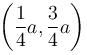Courses

# Differential Calculus MCQ Level - 2

## 10 Questions MCQ Test Topic wise Tests for IIT JAM Physics | Differential Calculus MCQ Level - 2

Description
This mock test of Differential Calculus MCQ Level - 2 for Physics helps you for every Physics entrance exam. This contains 10 Multiple Choice Questions for Physics Differential Calculus MCQ Level - 2 (mcq) to study with solutions a complete question bank. The solved questions answers in this Differential Calculus MCQ Level - 2 quiz give you a good mix of easy questions and tough questions. Physics students definitely take this Differential Calculus MCQ Level - 2 exercise for a better result in the exam. You can find other Differential Calculus MCQ Level - 2 extra questions, long questions & short questions for Physics on EduRev as well by searching above.
QUESTION: 1

### The maximum value of x2 log (1/x) is :

Solution: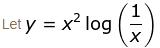x2(log 1 – log x)
= –x2  log x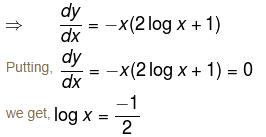or x e–1/2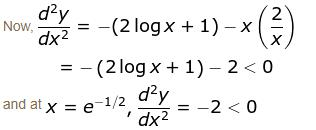Hence, y is maximum at x = 1/√e

QUESTION: 2

### The critical point of the function f(x) = (x – 1)2 + 1 from the following graph is :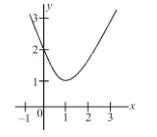Solution:

Turning point is (1, 1) as on one side of (1, 1), the curve is decreasing while the other, is increasing.

QUESTION: 3

### If for the twice differentiable function, f'(x) = f ''(x) = 0, x is

Solution:

If f'(x) = f''(x) = 0, then to tell whether x is a point of maxima or minima, we need to know the state of all its higher derivatives.

The correct answer is: can’t say

QUESTION: 4

The function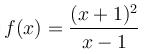at the point (3, 8) has :

Solution: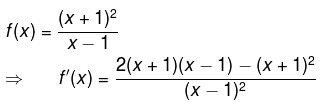As we can see that the denominator in f'(x) will always be positive.

Also, we notice that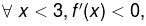i.e., f is a decreasing function and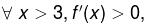i.e., f is an increasing function.

Hence, we conclude that there must be minima at x = 3.

QUESTION: 5

The function sin x(1 + cos x) at x = π/3  is :

Solution:

Let  f(x) = sin x(1 + cosx)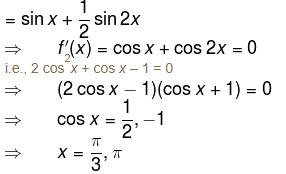Now, f''(x) = –sin x – 2 sin 2x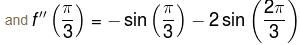= –1 < 0
Hence, f(x) will be maximum at x = π/3.

QUESTION: 6

The critical points of the function f(x) = (x – 1)(x – 2)2 in the interval [1, 2) :

Solution:

Let f(x) = (x – 1)(x – 2)2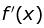'= (x – 2)2 + 2(x – 1)(x – 2)
= (x – 2)(3x – 4)
Putting= (x – 2)(3x – 4) = 0

We get,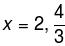∴ The only critical point is x = 4/3 as critical points always lie inside the interval.

The correct answer is: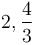QUESTION: 7

The turning points of the function y = x3 – 3x + 2 are :

Solution:

f(x) = x3 – 3x + 2
Putting f'(x) = 3x2 – 3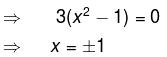We get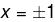as the critical or turning points.
The correct answer is: –1 and +1

QUESTION: 8

The absolute maximum and absolute minimum value of the function f(x) = x3 – 3x2 + 1 for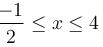are :

Solution:

f(x) = x3 – 3x2 + 1
⇒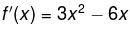Putting f'(x) = 0,
i.e., 3x2 – 6x = 0
3x(x – 2) = 0
⇒ x = 0, 2
Now, f''(x) = 6x – 6
f''(0) = –6 < 0
and f''(0) = 6 > 0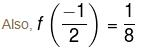f(4) = 17
f(0) = 1
f(2) = –3
Hence, absolute maxima will be 17 (at x = 4)
and hence absolute minima will be –3 (at x = 2).

The correct answer is: 17, –3

QUESTION: 9

The critical points of f(x) = x3/5(4 – x) are :

Solution:

f(x)=x3/5(4 – x)
= 4x3/5 + x8/5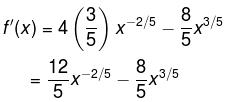Putting f'(x) = 0,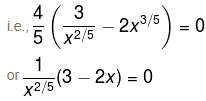Now, either, 3 – 2x = 0  ⇒ x = 3/2 is the critical point or x = 0 (at x = 0, f'(x) doesn’t exist)

The correct answer is:  3/2 and 0

QUESTION: 10

Two numbers whose sum is a, if the product of one by the cube of the other is to be maximum. Then the numbers are :

Solution:

Let the two numbers be x and y
⇒ x + y = a
or      x = a – y
We need to maximise, z = xy3
or  z = (a – y)y3
ay3 – y4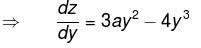Putting dz/dy = 0, i.e
3ay2 – 4y3 = 0
or y(3a – 4y) = 0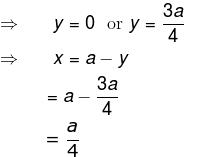The correct answer is: